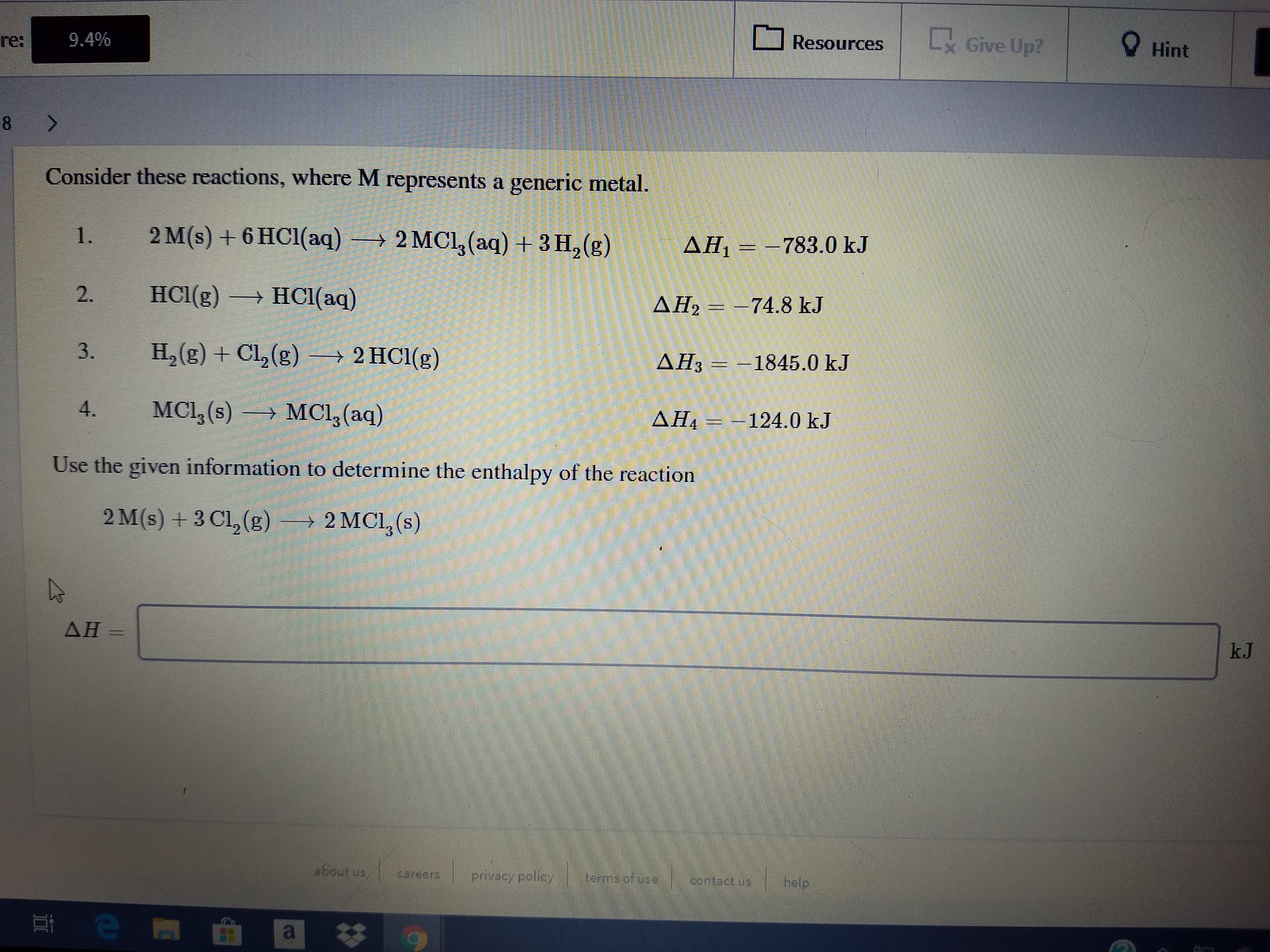# Give Up?O Resources9.4%re:Hint8 >Consider these reactions, where M represents a generic metal.2 M(s) + 6 HC1(aq) → 2 MCl,(aq) + 3 H, (g)1.AĦ, = –783.0 kJHCI(g)HCl(aq)→AH2 = –74.8 kJH,(g) + Cl, (g) → 2 HCl(g)AH3 = –1845.0 kJMCI, (s) → MCl, (aq)4.AH -124.0 kJUse the given information to determine the enthalpy of the reaction2 M(s) + 3 Cl,(g)→ 2 MCl, (s)S.AHkJabout usprivacy policyterme of usecontact.uahelpen2.3.

Question
41 viewshelp_outlineImage TranscriptioncloseGive Up? O Resources 9.4% re: Hint 8 > Consider these reactions, where M represents a generic metal. 2 M(s) + 6 HC1(aq) → 2 MCl,(aq) + 3 H, (g) 1. AĦ, = –783.0 kJ HCI(g) HCl(aq) → AH2 = –74.8 kJ H,(g) + Cl, (g) → 2 HCl(g) AH3 = –1845.0 kJ MCI, (s) → MCl, (aq) 4. AH -124.0 kJ Use the given information to determine the enthalpy of the reaction 2 M(s) + 3 Cl,(g) → 2 MCl, (s) S. AH kJ about us privacy policy terme of use contact.ua help en 2. 3. fullscreen
check_circle

Step 1

The Hess's law can be described as the law that will be used to calculate the value of the change in enthalpy for the net chemical reaction will be equivalent to the addition of all the change in enthalpy of the single chemical reactions into which the net chemical reaction will be divided.

Step 2

The equation for the reaction (1) of M and HCl is shown below:

Step 3

The equation for the reactio...

### Want to see the full answer?

See Solution

#### Want to see this answer and more?

Solutions are written by subject experts who are available 24/7. Questions are typically answered within 1 hour.*

See Solution
*Response times may vary by subject and question.
Tagged in

### Physical Chemistry